Courses

# Test: Arithmetic - 2 (2018-2017)

## 20 Questions MCQ Test UPSC Topic Wise Previous Year Questions | Test: Arithmetic - 2 (2018-2017)

Description
This mock test of Test: Arithmetic - 2 (2018-2017) for UPSC helps you for every UPSC entrance exam. This contains 20 Multiple Choice Questions for UPSC Test: Arithmetic - 2 (2018-2017) (mcq) to study with solutions a complete question bank. The solved questions answers in this Test: Arithmetic - 2 (2018-2017) quiz give you a good mix of easy questions and tough questions. UPSC students definitely take this Test: Arithmetic - 2 (2018-2017) exercise for a better result in the exam. You can find other Test: Arithmetic - 2 (2018-2017) extra questions, long questions & short questions for UPSC on EduRev as well by searching above.
QUESTION: 1

### X and Y are natural numbers other than 1, and Y is greater than X. Which of the following represents the largest number? 

Solution:

Let X = 6, Y = 8, then
(a) 48
(b) 3/4
(c) 4/3
(d) 7/24
Hence X.Y is largest number

QUESTION: 2

### If X is between – 3 and – 1, and y is between – 1 and 1, then X2 - Y2 is in between which of the following? 

Solution:

–3 < X < –1    ⇒ 1 < X2 < 9
–1 < Y < 1      ⇒ 0 < Y2 < 1
Min (X2 – Y2) = min (X2) – max (Y2) = 1 – 1 = 0
Max (X2 – Y2) = max (X2) – min (Y2) = 9 – 0 = 9
∴ 0 < (X2 – Y2) < 9

QUESTION: 3

### 19 boys turn out for playing hockey. Of these, 11 are wearing hockey shirts and 14 are wearing hockey pants. There are no boys without shirts and/or pants. What is the number of boys wearing full uniform? 

Solution: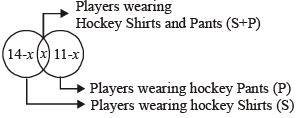Using venn diagram the given information can be represented as follows:
Let the number of players wearing both hockey shirt and pant be x
∴ n (st+1p) = x, n (s) = 14 – x
n (p) = 11 – x
n (T) = 19 (∴ T = Total number of players)
∴ n (T) = n (s) + n (p) + n (S + P)
∴ 19  = 14 – x + 11 –x + x
∴ 19 = 25 – x
∴ x = 25 – 19 = 6

QUESTION: 4

A student has to get 40% marks to pass in an examination. Suppose he gets 30 marks and fails by 30 marks, then what are the maximum marks in the examination?



Solution:

Total passing marks = 30 + 30 = 60
Percentage of passing marks = 40%
Let total marks be x
According to the question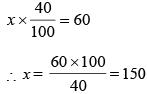QUESTION: 5

A bookseller sold ‘a’ number of Geography textbooks at the rate of ₹ x per book, ’a + 2’ number of History textbooks at the rate of ₹ (x + 2) per book and ‘a – 2’ number of mathematics textbooks at the rate of ₹ (x –2) per book. What is his total sale in ₹?



Solution:

Total price of geography textbooks = a × x = ₹ ax
Total price of History textbooks = (a + 2) (x + 2)
= ax + 2a + 2x + 4
Total Price of Mathematics text books (a – 2) (x – 2)
= ax – 2a – 2x + 4
Total price = ax + ax +2a + 2x + 4+ ax – 2a – 2x + 4 = 3ax + 8

QUESTION: 6

A number consists of three digits of which the middle one is zero and their sum is 4. If the number formed by interchanging the first number itself by 198, then the difference between the first and last digits is



Solution:

Let unit digit be x and hundred’s digit be y
Number = x × 1 + 0 × 10 + y × 100 = x + 100 y
Aste interchange of digits number = y × 1 + 100 × x
= 100 x + y
According to the question
100x + y – x – 100y = 198
99 (x – y) = 198
∴  x – y = 2
Hence difference = 2

QUESTION: 7

There are 24 equally spaced points lying on the circumference of a circle. What is the maximum number of equilateral triangles that can be drawn by taking sets of three points as the vertices?



Solution:

Each equilateral triangle is made by joining the three points as in the figure given below. Circle is divided into three sectors.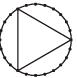Hence, required number of triangles = 24/3 = 8

QUESTION: 8

A shopkeeper sells an article at ₹ 40 and gets X% profit. However, when he sells it at ₹ 20, he faces same percentage of loss. What is the original cost of the article?



Solution:

Selling price of article = 40
Profit = x%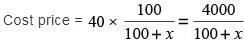Again, Selling price of article = 20
Loss = x%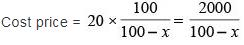Now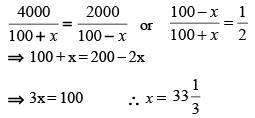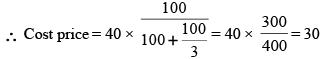QUESTION: 9

If x – y = 8, then which of the following must be true?
1. Both x and y must be positive for any value of x and y.
2. If x is positive, y must be negative for any value of x and y.
3. If x is negative, y must be positive for any value of x and y.
Select the correct answer using the code given below.



Solution:

Checking statement 1
x – y = 8
Both x and y must be positive for any value of x and y.
It is not true because negative values of x and y or positive x and negative y is also possible
Checking statement 2
If x is positive, y must be negative for any value of x and y.
It is also not true because positive values of x and y are also possible.
Checking statement 3
If x is negative, y must be positive for any value of x and y.
Here if x is negative then only negative value of y is possible.
Hence, neither 1 nor 2 nor 3

QUESTION: 10

A person bought a refrigerator worth ₹ 22,800 with 12.5% interest compounded yearly. At the end of first year he paid ₹ 8,650 and at the end of second year ₹ 9,125. How much will he have to pay at the end of third year to clear the debt?



Solution:

Amount to be paid after 1 year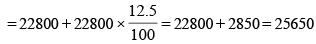He paid 8650 then amount left
= 25650 – 8650 = 17000
Amount to be paid after 2 years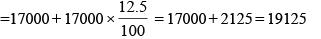He paid 9125 at the end of 2nd year then remaining amount = 19125 – 9125 = 10000
Amount to be paid at the end of 3rd year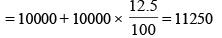QUESTION: 11

A lift has the capacity of 18 adults or 30 children. How many children can board the lift with 12 adults?



Solution:

18 Adults = 30 children (given)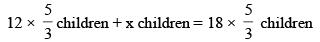x = 10 children

QUESTION: 12

Two walls and a ceiling of a room meet at right angles at a point P. A fly is in the air 1 m from one wall, 8 m from the other wall and 9 m from the point P. How many meters is the fly from the ceiling?



Solution: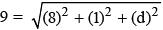⇒ 81 = 64 + 1 + (d)2
⇒ (d)2 = 16
d = 4.

QUESTION: 13

The monthly incomes of X and Y are in the ratio of 4 : 3 and their monthly expenses are in the ratio of 3 : 2. However, each saves ₹ 6,000 per month. What is their total monthly income?



Solution:

Let the monthly incomes of x and y be 4x and 3x.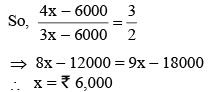Total income of x and y = (3 + 4)x
= ₹ 6,000 × 7 = ₹ 42,000

QUESTION: 14

A 2-digit number is reversed. The larger of the two numbers is divided by the smaller one. What is the largest possible remainder



Solution:

94 divided by 49 leaves the largest remainder 45.

QUESTION: 15

There are certain 2-digit numbers. The difference between the number and the one obtained on reversing it is always 27. How many such maximum 2-digit numbers are there?



Solution:

Let the two digit numbers are of the form ab.
So, 10a + b – (10b + a) = 27
⇒ 9a – 9b = 27
⇒ a – b = 3
(a, b) ⇒ (9, 6), (8, 5), (7, 4) (6, 3) (4,1), (5, 2)
Hence, 96 , 85 , 74 , 63 , 41, 52 and  25, 14, 36, 47, 58, 69 are the required numbers.

QUESTION: 16

P works thrice as fast as Q, whereas P and Q together can work four times as fast as R. If P, Q and R together work on a job, in what ratio should they share the earnings?



Solution:

Let the work done by Q in 1 day = x units.
So the work done by P in 1 day = 3x units
Work done by R in 1 day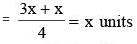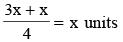Hence, the ratio of earnings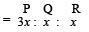= 3 : 1 : 1.

QUESTION: 17

The age of Mr. X last year was the square of a number and it would be the cube of a number next year. What is the least number of years he must wait for his age to become the cube of a number again?



Solution:

Mr. x’s present age 26, because 25 was a perfect square and the next year would be a perfect cube.
Next cube number → 64
Hence, minimum years required = 64 – 26 = 38 years.

QUESTION: 18

If for a sample data
Mean < Median < Mode
then the distribution is



Solution:
QUESTION: 19

How many numbers are there between 99 and 1000 such that the digit 8 occupies the units place?



Solution:

—  — 8
There are 9 values (1 to 9) for hundreds place digit.
While 10 values (0 to 10) for ten’s place digit.
Hence, 9 × 10 = 90 Numbers.

QUESTION: 20

Certain 3-digit numbers have the following characteristics:
1. All the three digits are different.
2. The number is divisible by 7.
3. The number on reversing the digits is also divisible by 7.
How many such 3-digit numbers are there?



Solution:

Let the numbers are of the form abc.
So, According to question,
100 a + 10b + c = 7K ....(i)
100c + 10b + a = 7m .....(ii)
From, (i) – (ii)
99a – 99c = 7 (k–m)
99 (a – c) = 7n
a – c = 7
a = 9, c = 2
a = 8, c = 1
Hence, 4 numbers, 259, 952, 168 and 861.